Disclosure: This post may contain affiliate links, meaning when you click the links and make a purchase, we receive a commission.

# Running Average: How to Calculate Using Excel’s Average(…) Function

In this post, you will learn how to calculate running average of a data set using Excel’s Average(…) function.

A business generates huge amount of data every year. Most of the data are related to time. One useful way to analyze your data is to find the average.

For example, assume that you run a store in your town. And you keep a record of your customers:

1. How many customers come to your store every month,
2. And how many times a customer comes to your store.

Or if you run a website or blog like me, then you will count how many visitors are coming to your blog every month.

These are just two examples; your business or website generates many more kind of data.

In this article, we shall learn how to calculate the running average of some values.

## Calculate Running Average

Let’s explain what running average is with an example. In the image below, you are seeing an Excel workbook titled Running Average. In the Visitors worksheet, you are seeing some data. Under Month column, there are twelve months: from Jan to Dec. Under visitors in my blog column, you are seeing the total number of visitors coming to my blog every month. The blog visitors have increased almost three times in the month of November. In Jan it was around 3 thousands and in the month of November it is around 8 thousands and 500 hundreds.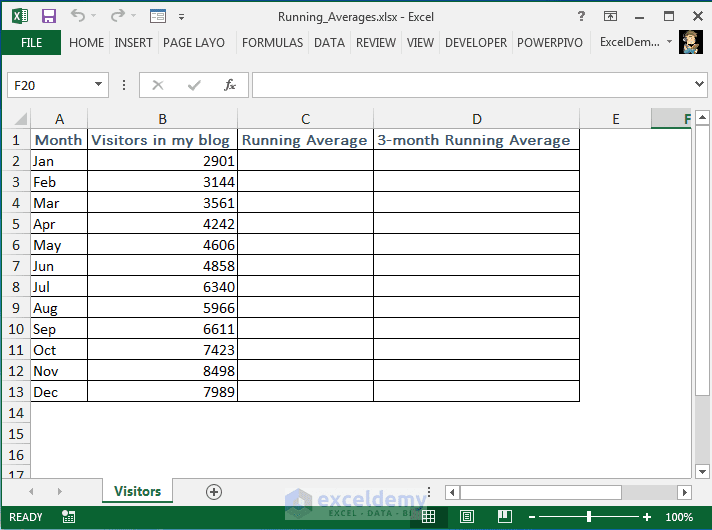Visitors came to my blog every month in Year 2015

At first we shall find out the running average of these values and then will find out the 3-month running average of these values. Then we shall talk about the differences of these two running averages.

Okay let’s find out the regular running average at first.

1. Select cell C2 and insert the average function, select cell B2 as the argument of the Average function, I am going to make it B2 reference as an absolute reference, so press F4 key in your keyboard, cell reference B2 turns into an absolute cell reference.
2. Then insert range operator and again insert cell reference B2 and finally place the closing parenthesis after the formula.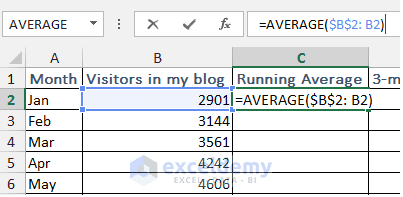Running average formula building…

3. Now I press return. The formula returns value 2901. This formula actually finds out the average of cell B2.
4. Now I select the cell and then copy the formula for the next cell. The formula returns 3022.5. I select the cell and click on the formula bar. You see this formula selects cells B2 to B3. So the formula calculates the average of cells B2 to B3. I am sure you are getting idea how running average actually works.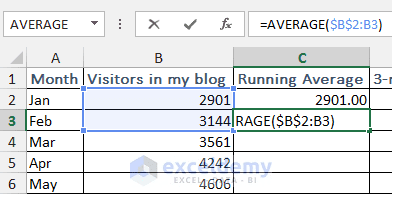Running average of first 2 values.

5. Now I copy the formula for other cells in the column. I select the last cell and click on the formula bar. You see this formula works on all the cells in the column, from cells B2 to B13.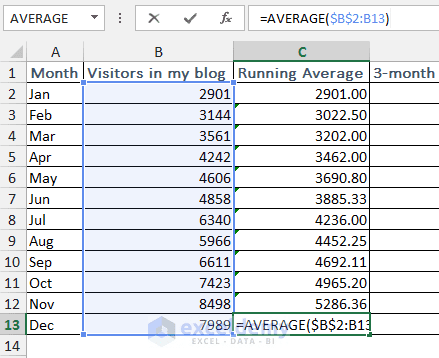Running average formula calculates running average of last 12 months.

So, this is how you can calculate running average of some values.

Now I shall show how you can calculate the 3-month running average.

1. Under 3-month running average column, I select the third cell D4. As we are going to find out the running average of 3 months, so we have to start from the third month. You cannot find out 3-month running averages using only 1 or 2 values.
2. I insert average function in cell D4, I select cells B2 to B4. This time I am not going to turn any cell references to absolute as when I shall copy this formula to the cells below, I want that for every formula it will select the last three values. So I close the function and press Enter.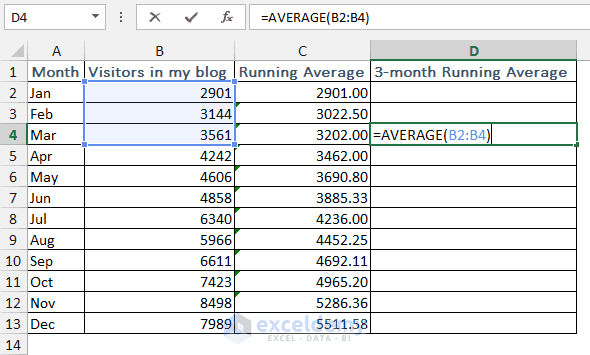3-Month Running Average Formula Building

3. The formula returns 3202. It is same as this running average. The reason is this running average finds out the average of these three values and this 3-month running average also do the same.
4. Now I copy the formula to other cells in the column. You get all 3-month running average.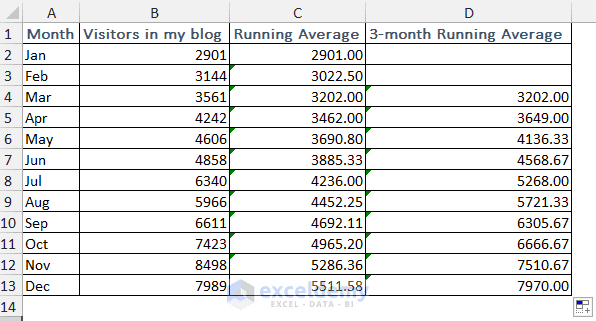3-Month Running Averages

Now let’s come to the point. What you say, which running average is showing the more practical scenarios? The regular running average or the 3-month running average? As a blogger what should my expected number of visitors in the month of Jan in year 2016? Is it 5511 or 7970?

Of course I will expect at least 8000 visitors will come to my blog in the month of January 2016, not 5511. 5511 is actually showing the result based on the last 12 months. And 7970 is actually showing the result based on the last 3 months. It is more practical.

You might ask yourself then which average you should use. My answer will be both. If you calculate both type of running averages for your data, you will get two different looks of your data.

This is a sample lecture of my course: Data Analysis in Excel with Statistics: Get Meanings of Data where you will learn Data Analysis with 52 case studies, problems, and their solutions!

1. Reply